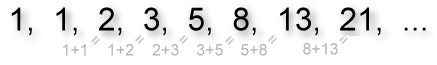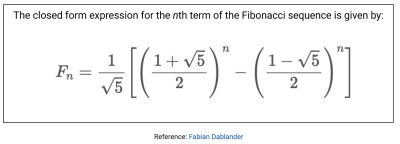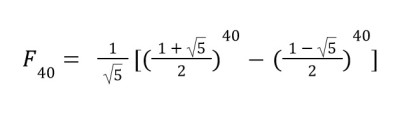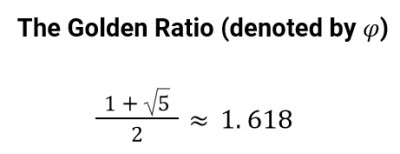# What Is The Fibonacci Sequence? (3 Key Ideas)

Perhaps you remember problems like the following from standardized tests:

Find the next two numbers in the given sequences:

• {3, 7, 11, 15, ___ , ____ }
• {1, 5, 25, 125, ___ , ____ }

Do these problems seem familiar? To solve them, we can look for patterns.

We can see from part a) that the pattern is “add 4,” so the next two terms are 19 and 23 (this is an arithmetic sequence – you can learn more here).

For part b) we can see that each term is a power of 5 so the next two terms are 54 = 625 and 55 = 3,125 (this is a geometric sequence – you can learn more here).

Those weren’t too bad. Let’s look at another sequence. What are the next two terms for this sequence?

• {0, 1, 1, 2, 3, 5, 8, 13, ___ , ____ }

It may not be as easy to see the pattern. Perhaps you’ve already noticed the pattern – we’ll discuss that in a bit.

In any case, this sequence is called the Fibonacci sequence. In this article, we’ll look at Fibonacci’s sequence and explore some real life situations in which the Fibonacci sequence occurs.

Python Fibonacci Series Using Loop ...
Python Fibonacci Series Using Loop and Recursion

Let’s get started.

First let’s talk about Fibonacci himself. Who was Fibonacci? And why does he have a special sequence named after him?

## Who was Fibonacci?

Fibonacci was an Italian mathematician, born around 1170. He is also known by the name Leonardo Pisano which means Leonardo of Pisa.

His original name, however, was Leonardo Fibonacci and this is how we know him. Fibonacci contributed quite a bit to number theory and published an important work in 1202 called Liber Abaci, which means, “Book of the Abacus.” Despite his important works, he is mainly known for the famous sequence!

## How did Fibonacci discover this sequence?

Curiously, Fibonacci considered a rabbit problem to develop this sequence. The situation went like this:

Suppose a male and female rabbit are put into a field together. At the end of one month, they mate but there is still only one pair. At the end of the second month, the female produces another male and female so now there are two pairs of rabbits. Suppose that the female always produces one new pair – one male, one female – every month from the second month on.

Leonardo Fibonacci

Fibonacci asked this question:

• How many pairs of rabbits will there be in one year?

Let’s summarize how many pairs of rabbits we’ll have at the end of each month.

• Beginning of First Month: 1 pair
• End of First Month: 1 pair
• End of Second Month: 2 pairs – the original pair of rabbits PLUS the pair the female produced
• End of Third Month: 3 pairs – the original female produces another pair so now we have 3 pairs
• End of Fourth Month: 5 pairs – the original female produces another pair, making 4 pairs AND the female from the end of the second month produces her first pair of rabbits, making 5 pairs total

We start to see a pattern. This pattern is what’s called the Fibonacci sequence. The sequence technically starts with the number 0 but from there we can use the rabbit problem to generate these numbers.

Fibonacci Sequence:  {0, 1, 1, 2, 3, 5, 8, 13, 21, 34, 55, 89, 144 …}

Do you notice the pattern? Instead of trying to figure out the number of rabbits we’ll have at the end of a certain month, we may notice that to generate the next number of the sequence, we can add the previous two terms.To get the next term in the Fibonacci, sequence, we add the previous two terms.

To answer Fibonacci’s original question – there will be 144 rabbits at the end of one year!

This, of course, assumes a few unlikely ideas – none of the rabbits die and the female rabbits always produce a male and female pair. In any case, it’s a pretty interesting problem! And, that’s a LOT of rabbits!

What if we wanted to write a formula that would generate the Fibonacci sequence?

As we saw, we can add the previous two terms to get the next term in the sequence. It’s easier to define the sequence using a recursive formula.

A recursive formula defines the sequence by one or more of the previous terms and includes the first term (or the first few terms) of the sequence.

Recursive formulas have limitations however. Suppose someone asks the question, “What is the 40th term of the Fibonacci sequence?” This will be difficult to answer with a recursive formula since we need to know the value of the 38th and 39th terms of the sequence!

It would be helpful to have a closed form expression for the nth term of the Fibonacci sequence. A closed form expression allows us to find any term in the sequence by calculating the term directly.

Unlike a recursive definition, a closed form expression doesn’t rely on the previous term or terms. A closed form expression exists for the Fibonacci sequence but it’s a bit difficult to work with. Let’s have a look:This is the closed form of the Fibonacci sequence, which works for any value of n (n must be a positive integer).

Suppose we want to find the 40th term of the Fibonacci sequence. We can used the closed form expression to calculate this:This is the closed form for the nth Fibonacci term with n = 40 substituted.

It’s definitely helpful to have a calculator for this one! Be aware of the order of operations. From the calculator, we obtain 102,334,155.

## Why is the Fibonacci so important?

Fibonacci number sequences come up in all kinds of situations.

“The Fibonacci number sequence describes how things grow, and also how they decay. It can predict the increase in the population of a colony of rabbits, and of a colony of bacteria. It also predicts the way that a colony that overwhelms its resources will decline. Fibonacci numbers are also used to help predict occurrences in the financial markets.”

Reference: actforlibraries

In nature, the Fibonacci Sequence occurs frequently. If you simply search on “Fibonacci sequences nature,” you’ll return lots of results! Here’s an interesting find from Mathnasium.com:

The Golden Ratio

An interesting pattern of the Fibonacci sequence is that if we take any two successive terms in the sequence and divide them, we obtain a number that is close to the Golden Ratio. Have you heard of the Golden Ratio? If not, here is a refresher:The golden ratio involves a radical that is a non-terminating decimal (the square root of 5).

“The Greeks also had observed that the Golden ratio provided the most aesthetically pleasing proportion of sides of a rectangle.” A rectangle with sides in this proportion is called a Golden Rectangle.

There is a special relationship between the Fibonacci sequence and the Golden Ratio. Let’s look a bit closer at our sequence. We’ll list the Fibonacci sequence starting with 2 in the first column, then we’ll start with 3 in the second column. Divide the numbers in the second column by the numbers in the first column and we obtain a ratio that seems to have a limiting value.

As we continue to divide successive terms in the Fibonacci sequence, the quotient gets closer and closer to the Golden Ratio!

In fact, the limit of the ratio of successive terms (greater term divided by smaller term) is equal to the Golden Ratio! So, the Fibonacci sequence and the Golden Ratio go hand in hand.

## The Fibonacci sequence in music and art

Have you heard of a Stradivarius violin? Antonio Stradivari (1644-1737), the Italian violin maker, made some of the most beautiful and best sounding violins in the world.

How did he do this? In addition to studying under the tutelage of master luthiers (craftspeople who build and repair stringed instruments with a neck and soundbox) and his natural genius, he also incorporated the Fibonacci sequence and the Golden Ratio into his violins’ designs.

In the diagram below, you can see that the ratio of various lengths of the parts of the violin are in the Golden Ratio. Pretty cool, right?

Notice that the piano also uses Fibonacci numbers:

Composers and artists have been incorporating the Fibonacci sequence into their works for ages. According to Classic FM, Mozart and Lady Gaga both used the Fibonacci sequence in their compositions!

## What is a good visual for the Fibonacci sequence?

Often the Fibonacci sequence is shown as a spiral-like shape, as in the image below. The spiral is generated by partitioning a golden rectangle!

In the image, each colored square has an area equal to the number in the square. Notice the sequence actually starts with the two small squares, each with area 1, then the square with area 2, then the square with area 3, although those numbers aren’t shown in the picture.

This resulting spiral that’s drawn is called the Fibonacci Spiral.

This spiral also occurs in nature! Look at the following examples: